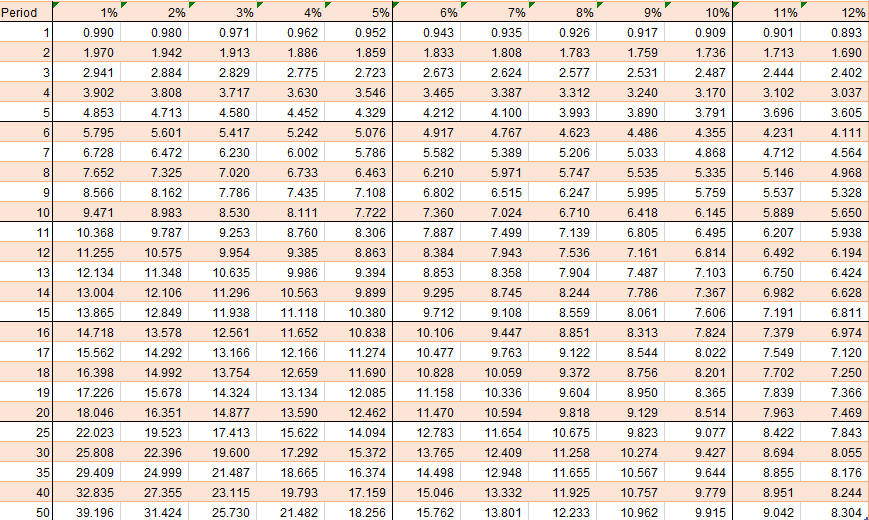# Present Value Annuity |Table | Formulas | Calculator

## What Is An Annuity?

An annuity is a steady stream of payments set over a set interval.

A common example of an annuity is a retirement plan where the investor purchased the annuity and at a point in the future, the retirement fund pays the investor a set amount each month. There are ordinary annuities where payments occur at the end of the period and present value of an annuity due or PVAD where the payments occur at the beginning of the period.

## How To Calculate An Annuity?

To calculate the value of an annuity you use an interest rate to discount the amount of the annuity. The interest rate can be based on a number of factors such as expected return on investments, cost of capital or other factors.

To find the value of the annuity, an annuity table or annuity calculator is used to determine the present value of an annuity. The annuity table looks at the number of equal payments or series of payments made over time discounted by rates of interest.

Multiplying the number of payments by the discount rate, the payment amount is calculated.

## Present Value Annuity Formula

The present value annuity factor is based on the time value of money. The time value of money is a concept where waiting to receive a dollar in the future is worth less than a dollar today, since a dollar today could be invested and be worth more in the future. Because of this, we need a way to compute the present value of future cash flows.

The present value of an annuity formula is as follows:

P = PMT [(1 – (1 / (1 + r)n)) / r]

P = Present value of the annuity
PMT = The amount of each annuity payment
r = Interest rate
n = Number of periods

## Present Value Factor for an Ordinary Annuity Table

This table can be used to calculate the present and future value of annuity. The present value formula is handy, but it can be faster to compute the value using an annuity table or a present value of annuity calculator. In the left vertical column you have the time period. The top horizontal column is the interest rate. The numbers in the middle are the annuity factor.

To find the present value, the following example may help. If an investor wanted to purchase a \$100,000 annuity providing 20 payments at 8%, we would look at the intersection of 20 and 8% in the table to get the factor of 9.818. To arrive at the present value of the annuity, you would multiple 9.818 by \$100,000 to arrive at the present value of \$981,800.